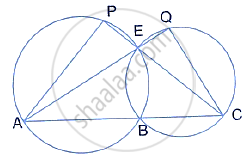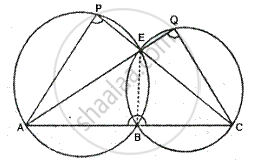Share

In the Given Figure; Abc, Aeq and Cep Are Straight Lines. Show that ∠Ape and ∠Cqe Are Supplementary. - ICSE Class 10 - Mathematics

ConceptTangent Properties - If Two Circles Touch, the Point of Contact Lies on the Straight Line Joining Their Centers

Question

In the given figure; ABC, AEQ and CEP are straight lines. Show that ∠APE and ∠CQE are supplementary.SolutionGiven – In the figure, ABC, AEQ and CEP are straight line
To prove - ∠APE + ∠CQE = 180
Construction – join EB
Proof – in cyclic quad ABEP,
∠APE + ∠ABE = 180°      ………. (1)

Similarly, in cyclic quad BCQE
∠CQE + ∠CBE = 180°        ………. (2)
Adding (1) and (2),
∠APE + ∠ABE + ∠CQE + ∠CBE = 180° + 180° = 360°
⇒ ∠APE +∠ABE + ∠CBE = 360°
But, ∠ABE + ∠CBE = 180°     [Linear pair]

∠APE + ∠CQE + 180° = 360°
⇒ ∠APE + ∠CQE = 360° -180° = 180°
Hence ∠APE and ∠CQE are supplementary.

Is there an error in this question or solution?
Solution In the Given Figure; Abc, Aeq and Cep Are Straight Lines. Show that ∠Ape and ∠Cqe Are Supplementary. Concept: Tangent Properties - If Two Circles Touch, the Point of Contact Lies on the Straight Line Joining Their Centers.
S